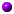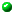Course webpage for Freshman Seminar 24i: Mathematical Problem Solving (Fall 2010)

If you find a mistake, omission, etc., please let me know by e-mail.

Here's a clearer picture of the background pattern...
September 1
Initial meeting:
• Introductions, à la Pál Erdös: “What's your name and what's your problem?”
(from our e-mail correspondence; see the list above, plus Iurie on an alternating sum of interval lengths when [0,mn] is divided both into m and into n equal parts, me on sphere packings, and some more details on some of your favorites)
• Expectations, and organizational / administrative details
• Some initial problems to think about: one 5x5 and two 7x7 Kenken puzzles; which of 51/2 + 131/2 and 341/2 is larger?

September 13

• Various approaches to 51/2 + 131/2 vs. 341/2. How were these numbers 5, 13, 34 chosen? Generalizations and connections: factoring x2+1 or x2-1; Fibonacci numbers; the general formula for a1/2 + b1/2 vs. c1/2 via the sign of D(a,b,c) := a2 + b2 + c2 - 2 (ab + ac + bc), with unexpected extra symmetry, in turn leading us to Heron's formula for the area of a triangle of sides a1/2, b1/2, c1/2 (if it exists); we know how to make D(a,b,c)=4 or -4, but can we make |D(a,b,c)| even smaller (but not zero) for some natural numbers a,b,c?This turns out to be related with our CA Iurie Boreico's HCMR article on what was then his favorite problem — which as it happens refers to the “favorite problem” article of Zach Abel (who CA'd the '09 and '08 editions of this seminar) in earlier HCMR issues. Iurie's article gets rather technical, but at least some of the formulas in the “Preliminary Analysis” section should look familiar…
• Some more Kenken: last week's solution; parity tricks
• General considerations I: Where do problems come from, and (how and) why are they created? The uses of problems, to proposers and solvers, ranging from external applications to the satisfaction of solving a stumper; who is playing the “game” (proposer? other solvers? audience for solution?), and how this and other aspects of the context of the problem might influence one's strategy for solving (and presenting) the solution

September 20

• Progress report on small nonzero |D(a,b,c)| values: D(a,b,c) = 1 can happen! Almost trivially at first, with (a,b,c) = (0,u,u+1); but from one solution we can get another by keep two of the variables the same solving the resulting quadratic equation for the third. This gets from (0,u,u+1) to (u,u+1,4u+2), and beyond. [In a few weeks we'll see another way to explain why (4u+2)1/2 is a bit larger than u1/2 + (u+1)1/2.]
Likewise D(a,b,c) = -3, starting from the silly-looking (1,1,1) [an equilateral triangle, of area sqrt(3)/4] to (1,1,3) [an isoceles triangle with angles of 30, 30, and 120 degrees], (1,3,7), (3,7,19), and beyond, giving increasingly sharp triangles, all with the same area sqrt(3)/4, that can be plotted on the triangular lattice, as the original (5,13,34) triangle can on the square lattice (see the next item).
This technique can be used for other problems, finding for instance infinitely many solutions of a2 + b2 + c2 = abc starting from the symmetrical (3,3,3). For equations of arbitrary degree (rather than just quadratic) this is related with Viète's formulas for the coefficients of a polynomial with known roots and leading coefficient.
• More on the triangle inequality. Proving it algebraically, in effect via
D( x12 + y12, x22 + y22, (x1+x2)2 + (y1+y2)2 ) = -4 (x1y2-x2y1)2
[sorry, HTML doesn't handle expressions like x12 as well as TeX...]. Along the way we find that
(x1x2+y1y2)2 ≤ (x12+y12) (x22+y22),
which is one way to state the 2-dimensional case of the Cauchy-Schwarz inequality, whose importance and ubiquity in mathematics are hard to overstate. Equality holds if and only if x1y2=x2y1, which is to say if and only if the vectors (x1, y1) and (x2, y2) are proportional. In 3 dimensions, we find with a little more work that
D( x12 + y12 + z12, x22 + y22 + z22, (x1+x2)2 + (y1+y2)2 + (z1+z2)2 )
equals -4 times
(x1y2-x2y1)2 + (y1z2-y2z1)2 + (z1x2-z2x1)2;
so we get the triangle inequality for vectors (x1, y1, z1) and (x2, y2, z2), with equality if and only if one is a nonnegative multiple of the other, and also three-dimensional Cauchy-Schwarz:
(x1x2+y1y2+z1z2)2 ≤ (x12+y12+z12) (x22+y22+z22)
with equality if and only if the vectors are proportional. To do it in general, either proceed in the same way, ending up with a sum of (n2-n)/2 squares [where did I get this count of (n2-n)/2?]; we'll soon see a less mystifying proof.
Some applications of the triangle inequality: reflection tricks (and the physics explanation of why this is called “reflection” to begin with); distances on the surface of a cube.
• Inequalities in general; different approaches to the maximum of x-x2 (a.k.a. xy if x+y=1, which reveals a symmetry between x and 1-x); when solving an inequality problem, always try to determine the equality condition. Introduction to the AM-GM inequality: the arithmetic mean (AM, a.k.a. average) of any list of positive numbers is at least as large as their geometric mean (GM), with equality if and only if all the numbers being averaged are the same. So, for example, can maximize xyz given xy+xz+yz without calculus.
September 27
• End of the |D(a,b,c)| story: because D(a,b,c) = (a+b+c)2 - 4 (ab + ac + bc), D is either a multiple of 4 or exceeds by 1 a multiple of 4 (in other words, D is “congruent to 0 or 1 mod 4”). Indeed if a+b+c is even then its square is clearly a multiple of 4, while if it's odd, say a+b+c=2k+1, then its square is 4k2+4k+1 = 4(k2+k) + 1 (indeed an odd square is always a mod 8, but that gives us nothing more here). Conversely, using our work thus far it is not hard to show that if d is 0 or 1 mod 4 then D(a,b,c) = d is possible, so at last we're basically done with this problem. (The condition on d was originally reached by solving D(a,b,c) = d as a quadratic equation in c.)
• The problem of finding the farthest point on the surface of a cube from an edge midpoint, or from almost any other point on the surface, is surprisingly delicate, and some problems that look very close to this are still unsolved. It turns out that the point farthest from an edge midpoint is neither the opposite midpoint, nor a far corner, nor halfway between the opposite midpoint and far corner (as one might first calculate), but a third of the way. This is farther than the midpoint by a factor of only 145/144, which comes out to 1.003466…
• Max/min problems continued: the biggest volume of a half- or fully-open box of given surface area; more uses of Cauchy-Schwarz; geometrical minimization problems, e.g. minimizing distance from point to plane, or sum of reciprocals of distances from a point inside a given triangle to its sides

October 4

• Cauchy-Schwarz, cont'd; e.g. a geometric minimization problem: giving a triangle, find point in the interior minimizing the sum of inverse distances to the three sides.
• Geometric solution of the problem of minimizing the sum of distances from a point to the three vertices of an acute triangle, leading to the Fermat point and some of the remarkable properties of an associated configuration of three equilateral triangles. Physical (vector field) interpretation. Students' suggested variations and generalizations (and some comments, e.g. the importance of homogeneity [a.k.a. “dimensional analysis”] — which is yet another example of exploiting the symmetry of a problem)
• The “quadrilateral inequality” encountered during the geometrical solution suggest a segue to the important technique of induction. Base case (Sn0), inductive hypothesis (Sk), inductive step (Sk implies Sk+1). Variation: “strong induction” (equivalently: apply induction to the statement
Sn: “Sk is true for each k between n0 and n”
rather than to Sn itself). Standard examples of induction problems: sums and and other recursions, once we've guessed the answer from the pattern of the first few examples (an important problem-solving technique in general, but beware of false trails such as
32+42=52,   33+43+53=63,  but   34+44+54+64≠74);
maximal number of slices of a pizza produced by n straight cuts.Here are some more induction problems

[October 11: no meeting — University holiday (Columbus Day)]

October 18 (abbreviated)

• Reviewing some of the induction problems; inductive construction of formula for 1k + 2k + 3k + … + nk (each k = 0, 1, 2, 3, …); the role of tools like the OEIS = Online Encyclopedia of Integer Sequences) in guessing the pattern (and getting more information)
• further example: the formula n! / (k! (n-k)!) for the k th entry of the n th row of Pascal's triangle, and some other interpretations
• Introduction to generatingfunctionology (yes, the single-word typesetting is a joke, but blame Wilf for it, not me), via Pascal's triangle again: (1+x)n as a generating polynomial for the nth row, proved by induction and used to re-explain the fact that the sum of all the entries in that row is 2n and to find the sum of their squares via the xn coefficient of (1+x)n (1+x)n = (1+x)2n.